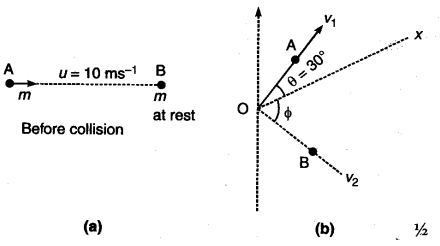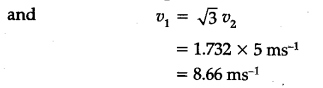# A and B are two particles having the same, mass tn. A is moving along x-axis with a speed of 10 n\${{m}^{-1}}\$, and B is at rest. After undergoing a perfectly elastic collision, with B, particle A gets scattered through an angle of 30°

A and B are two particles having the same, mass tn. A is moving along x-axis with a speed of 10 n\${{m}^{-1}}\$, and B is at rest. After undergoing a perfectly elastic collision, with B, particle A gets scattered through an angle of 30°. What is the direction of motion of B, and the speeds of A and B, after this collision ?